Learning Library
Who we are
Guidance

# Practice Writing the Alphabet

Kindergartners will practice writing both capital and lower case letters of the alphabet while thinking up words of their own that start with each letter.

Click on a worksheet in the set below to see more info or download the PDF.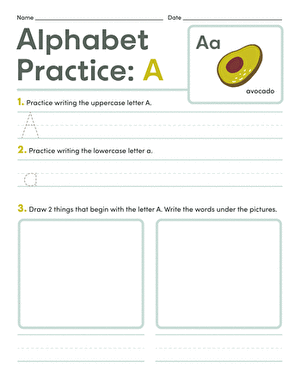### Alphabet Practice: A

Kindergarteners will become familiar with the letter A! Write the letter A in upper and lowercase form then draw and label two objects beginning with A.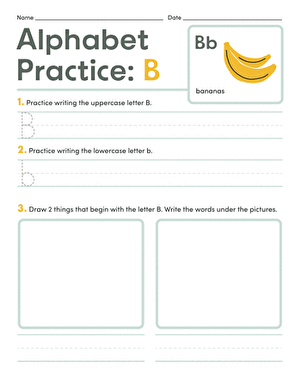### Alphabet Practice: B

Practice writing the letter B in the lines provided then draw and label two objects beginning with B.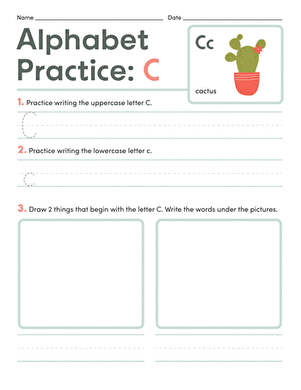### Alphabet Practice: C

Practice writing the letter C, then draw and label two objects that begin with C.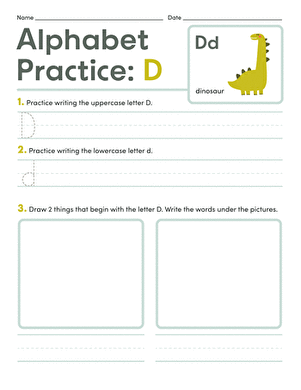### Alphabet Practice: D

Draw and label two objects beginning with the letter D then practice writing D's in the space provided.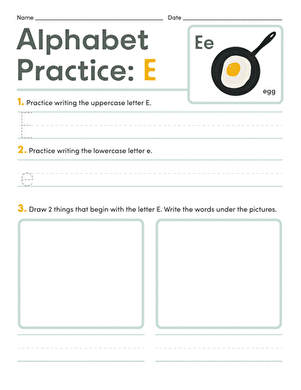### Alphabet Practice: E

Learn about the letter E in this alphabet worksheet! Practice writing the letter E then draw and label two objects beginning with the letter E.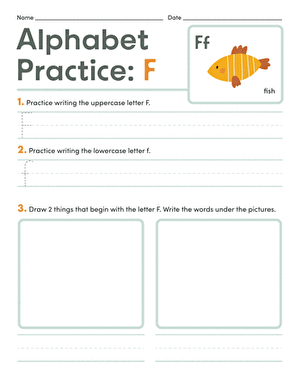### Alphabet Practice: F

F is for football! Practice writing the letter F then draw and label two items beginning with F.

The set is continued below.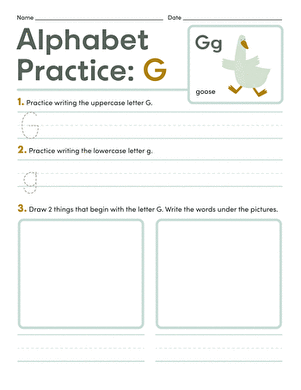### Alphabet Practice: G

G is for grapes! Practice writing the letter G then draw and label two objects beginning with G in the space provided.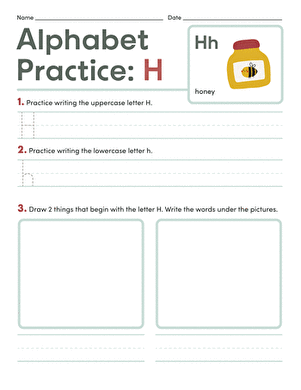### Alphabet Practice: H

H is for Hat! Practice writing the letter H then draw and label two objects beginning with H in the space provided.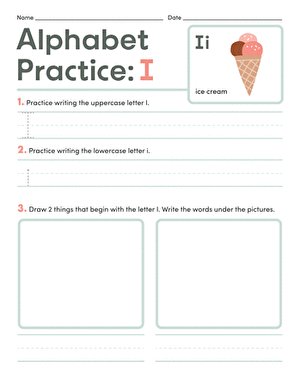### Alphabet Practice: I

Learn about the letter I by with this alphabet exercise! Write the letter I in uppercase and lowercase form then draw and label two objects beginning with I.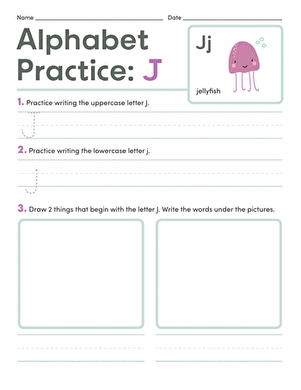### Alphabet Practice: J

Kindergarteners will jump at the chance to complete this fun alphabet exercise featuring the letter J.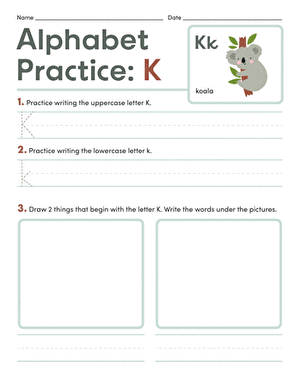### Alphabet Practice: K

Complete this alphabet exercise by practicing writing the letter K then drawing and labeling two objects that begin with K.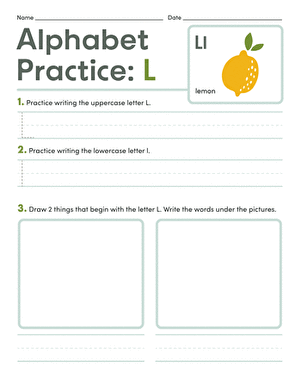### Alphabet Practice: L

Draw and label two objects beginning with the letter L then practice writing uppercase and lowercase L's!

The set is continued below.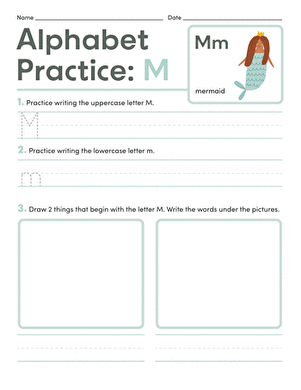### Alphabet Practice: M

Kindergarteners will have a magnificent time mastering the letter M by practicing writing M's then drawing and labeling two objects beginning with M!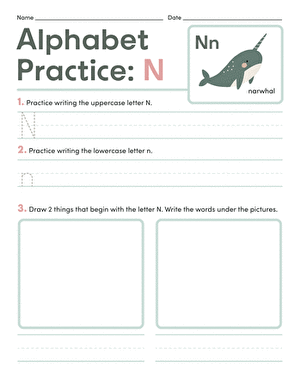### Alphabet Practice: N

Draw and label two objects beginning with the letter N in the space provided then practice writing N in lowercase and uppercase form.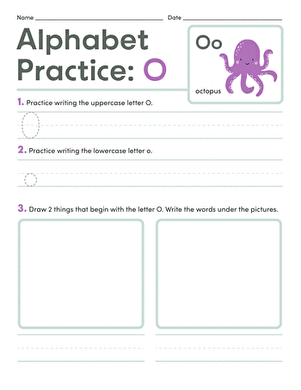### Alphabet Practice: O

Learn about the letter O in this fun alphabet worksheet! Begin by writing O in uppercase and lowercase form then draw and label two objects that start with O.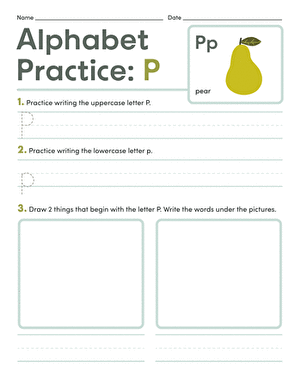### Alphabet Practice: P

Practice working with the letter P by writing it in lowercase and uppercase form then draw and label two objects beginning with P.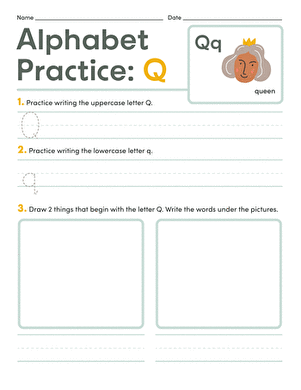### Alphabet Practice: Q

Q is for quarter! Think of two other objects beginning with Q then draw and label them. Next, practice writing the letter Q in lowercase and uppercase form.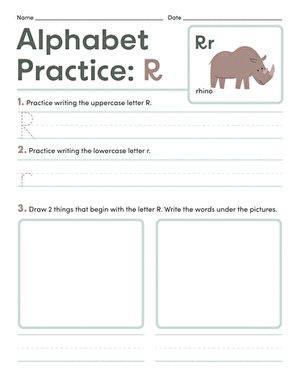### Alphabet Practice: R

Draw and label two objects beginning with the letter R then practice writing R's in lowercase and uppercase form.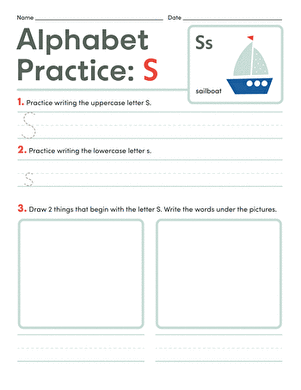### Alphabet Practice: S

Practice using the letter S by drawing and labeling two objects beginning with S and writing S's in lowercase and uppercase form.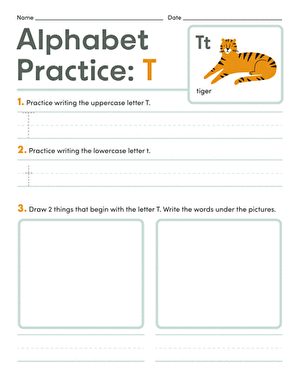### Alphabet Practice: T

T is for treasure! Practice writing the letter T in uppercase and lowercase form then draw and label two objects beginning with T.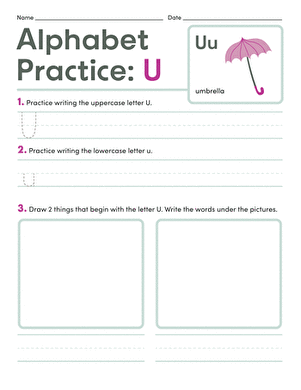### Alphabet Practice: U

Learn all about the letter U in this alphabet worksheet! Write U in both uppercase and lowercase form then draw and label two objects beginning with U.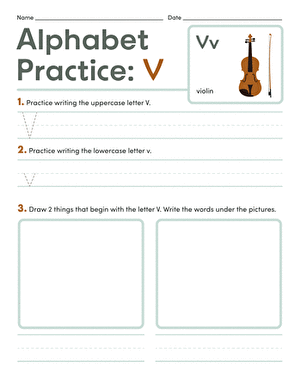### Alphabet Practice: V

Kindergarteners can practice writing V's in uppercase and lowercase form then draw and label two objects beginning with V.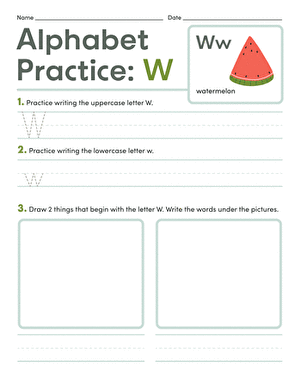### Alphabet Practice: W

Draw and label two objects beginning with the letter W then practice writing W's in both uppercase and lowercase form.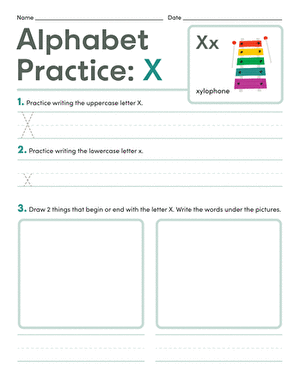### Alphabet Practice: X

Kindergarteners will be challenged to think of two objects beginning with the letter X then draw and label them. Get more practice by writing X's.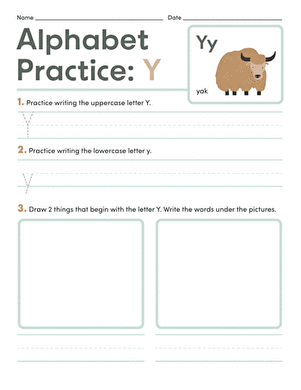### Alphabet Practice: Y

Kindergarteners can practice writing Y and gain additional practice with Y by drawing and labeling two objects beginning with Y.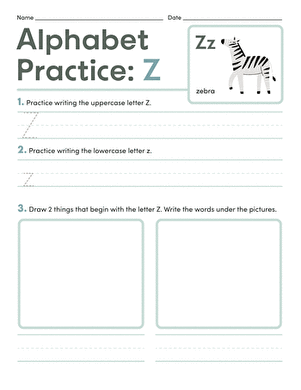### Alphabet Practice: Z

Think of two objects that begin with the letter Z and draw and label them. Then practice writing uppercase and lowercase Z's, too!